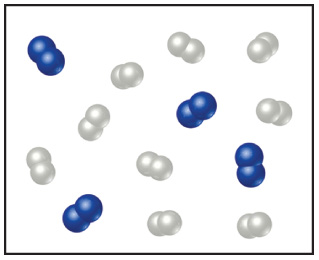# Problem: Nitrogen (N2) and hydrogen (H2) react to form ammonia (NH3). Consider the mixture of N2 and H2 shown in the accompanying diagram. The blue spheres represent N, and the white ones represent H.Write the balanced chemical equation for the reaction.

###### FREE Expert Solution

We’re asked to write the balanced chemical equation for the reaction of N2 and O2 based on the given diagram.

Recall that when balancing chemical equations, we have to make sure that the number of elements on both sides is equal with the lowest whole number coefficients.

The reactants are:

• 3 moles N2 (blue)
• 9 moles H(white)

The equation for the reaction can be written as:

N2 + H→ NH3  (not balanced)

**Write an arrow to separate products and reactants, use a plus sign for more than one reactant/product

83% (442 ratings)###### Problem Details

Nitrogen (N2) and hydrogen (H2) react to form ammonia (NH3). Consider the mixture of N2 and H2 shown in the accompanying diagram. The blue spheres represent N, and the white ones represent H.Write the balanced chemical equation for the reaction.